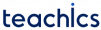# T Flip Flop

A T flip-flop is a modified form of the JK flip flop. It is called a toggle flip flop because of its toggling operation. T flip-flop is constructed by connecting the J and K inputs, creating a single input called T as shown in the following figure.

### Working of T Flip flop

The circuit diagram of a T flip-flop is given below:

When the clock is set to low, the output remains as it is whether the input signal is set to high or low.

When the clock is high, and the T signal is set to low(0), it will not affect the present state of the output and the response will not change. When the T signal is set to high(1), then it will invert the present output. That is, if the present output is high (1), it converts into low (0); if the present output is low (0), it converts it into high (1). It means it will toggle the output signal.

This operation is described in the following characteristics table and truth table.0 0 0
0 1 1
1 0 1
1 1 00 X1 01 1The equation forcan be derived from the excitation table using K-map as following.0 0 0
0 1 1
1 0 1
1 1 0

Subscribe
Notify of